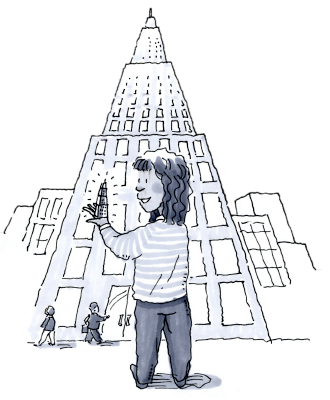### Home > CCAA > Chapter 9 Unit 8 > Lesson CC2: 9.2.3 > Problem9-79

9-79.

Glenda made a scale model of the Empire State Building in New York. The building itself is $1250$ feet high, and her scale model is $5$ feet high.

1. Write a ratio that compares the heights of the two buildings.

2. If the doorway of Glenda’s model is $2$ inches wide, how wide would you expect the doorway of the real building to be? Write and solve a proportion.

$41$ feet, $8$ inches. Remember to show your work.

3. If the real building contains $102$ floors, how many floors should Glenda’s model contain?

Do you change the number of floors or the size of each floor?Use the sliders in the eTool below to adjust the heights for the scale factor ratio.
Click the link at right for the full eTool version: